# NCERT Solutions For Class 10 Maths Chapter 11 Exercise 11.1

Go back to  'Constructions'

## Chapter 11 Ex.11.1 Question 1

Draw a line segment of length $$7.6 \,\rm{cm}$$ and divide it in the ratio $$5:8$$. Measure the two parts.

#### What is known?

Length of line segment and the ratio to be divided.

Construction

#### Reasoning:

• Draw the line segment of given length.
• Then draw another line which makes an acute angle with the given line.
• Divide the line into $$m + n$$ parts where $$m$$ and $$n$$ are the ratio given.
• Basic proportionality theorem states that, “If a straight line is drawn parallel to a side of a triangle, then it divides the other two sides proportionally".

#### Steps: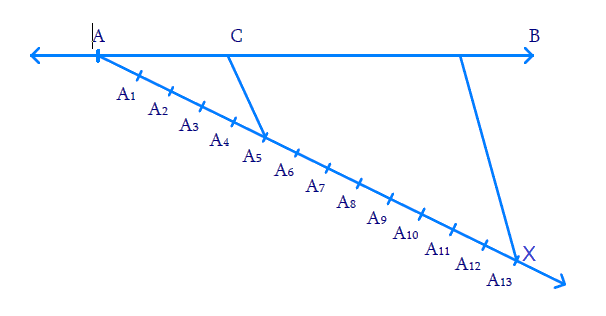(i) Draw $${AB = 7.6\, \rm{cm}}$$

(ii) Draw ray $$AX,$$ making an acute angle width $$AB.$$

(iii) Mark $$13\;(=\;5\;+\;8)$$ points $${A_1},\,{A_2},\,\ldots.\,{A_13}$$ on $$AX$$ such that$$\text{A}{{\text{A}}_{\text{1}}}\text{=}\,{{\text{A}}_{\text{1}}}{{\text{A}}_{\text{2}}}\text{=}{{\text{A}}_{2}}{{\text{A}}_{3}}\text{=}.....{{\text{A}}_{\text{12}}}{{\text{A}}_{\text{13}}}$$

(iv)  Join $${{BA}_{13}}$$

(v) Through $$A_5$$ (since we need $$5$$ parts to $$8$$ parts) draw  $${{C}}{{{A}}_{{5}}}$$ parallel to $${{B}}{{{A}}_{{{13}}}}$$ where $$C$$ lies on $$AB.$$

Now $${{AC: CB = 5:8}}$$

We find $$AC = 2.9 \,\rm{cm}$$ and $$CB = 4.7 \,\rm{cm}$$

Proof:

$${{C}}{{{A}}_{{5}}}$$ is parallel to $${{B}}{{{A}}_{{{13}}}}$$

By Basic Proportionality theorem, in $${{\Delta A}}{{{A}}_{{{13}}}}{{B}}$$

\begin{align}\frac{{AC}}{{CB}} = \frac{{{{A}}{{{A}}_{{5}}}}}{{{{{A}}_{{5}}}{{{A}}_{{{13}}}}}}{{ = }}\frac{{{5}}}{{{8}}}\,\,\end{align} (By Construction)

Thus, $$C$$ divides $$AB$$ in the ratio $$5:8$$.

## Chapter 11 Ex.11.1 Question 2

Construct a triangle of sides $$4 \;\rm{cm}$$, $$5 \;\rm{cm}$$ and $$6\; \rm{cm}$$ and then a triangle similar to it whose sides are \begin{align}\frac{2}{3}\end{align} of the corresponding sides of the first triangle.

#### What is known?

Sides of the triangle and the ratio of corresponding sides of $$2$$ triangles.

Construction.

#### Reasoning:

• Draw the line segment of largest length $$6\, \rm{cm}$$. Measure $$5 \;\rm{cm}$$ and $$4 \;\rm{cm}$$ separately and cut arcs from $$2$$ ends of the line segment such that they cross each other at one point. Connect this point from both the ends.
• Then draw another line which makes an acute angle with the given line ($$6\; \rm{cm}$$).
• Divide the line into $$(m + n)$$ parts where $$m$$ and $$n$$ are the ratio given.
• Two triangles are said to be similar if their corresponding angles are equal. They are said to satisfy Angle-Angle-Angle (AAA) Axiom.
• Basic proportionality theorem states that, “If a straight line is drawn parallel to a side of a triangle, then it divides the other two sides proportionally".

#### Steps: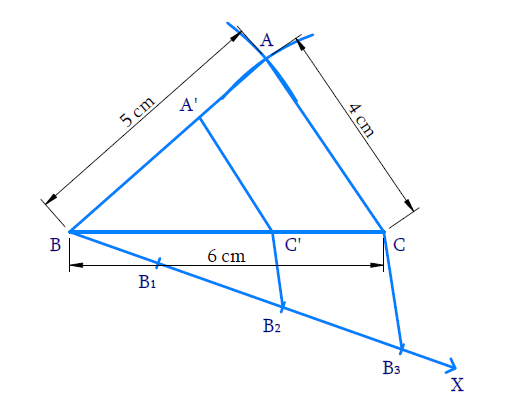Steps of constructions:

(i) Draw $$BC = 6 \text{cm.}$$ With $$B$$ and $$C$$ as centres and radii $$5 \;\rm{cm}$$ and $$4\,\rm{cm}$$ respectively draw arcs to intersect at $$A.$$  $${\rm{\Delta ABC}}$$ is obtained.

(ii) Draw ray $$BX$$ making an acute angle with $$BC.$$

(iii) Mark $$3$$ ( {$$3> 2$$ in the ratio \begin{align}\frac{2}{3}\end{align}points $${{{B}}_{{1}}}{{,}}\,\,{{{B}}_{{2}}}{{,}}\,\,{{{B}}_{{3}}}$$ on $$BX$$ such that $$\,{{B}}{{{B}}_{{1}}}{{ = }}{{{B}}_{{1}}}{{{B}}_{{2}}}{{ = }}{{{B}}_{{2}}}{{{B}}_{{3}}}$$ .

(iv) Join $$\,{{{B}}_{\rm{3}}}{{C}}$$ and draw the line through $${B_2}$$  (2nd point where $$2 < 3$$ in the ratio \begin{align}\frac{2}{3}\end{align}) parallel to $$B_3C$$ meeting $$BC$$ at $${{C'.}}$$

(v) Draw a line thorough $$\,{{C'}}$$ parallel to $$CA$$ to meet $$BA$$ at $$A’.$$ Now $$\,{{\Delta A'BC'}}$$ is the required triangle similar to $$\,{{\Delta ABC}}$$ where

\begin{align}\frac{{{{BC'}}}}{{{{BC}}}}&{{ = }}\frac{{{{BA'}}}}{{{\rm{BA}}}}{\rm{ = }}\frac{{{{C'A'}}}}{{{\rm{CA}}}}\\&{{ = }}\frac{{{2}}}{{{3}}}\end{align}

Proof:

In $$\Delta B B_{3} C, B_{2} C$$ is parallel to $$\,{{{B}}_{{3}}}{{C}} .$$

Hence by Basic proportionality theorem,

\begin{align}\frac{{{{{B}}_{{2}}}{{{B}}_{{2}}}}}{{{{B}}{{{B}}_{{2}}}}}&{{ = }}\frac{{{{C'C}}}}{{{{BC'}}}}\\&{{ = }}\frac{{{1}}}{{{2}}}\end{align}

Adding $$1$$,

\begin{align}\frac{{{{C'C}}}}{{{{BC'}}}}{{ + 1}}& = \frac{{{1}}}{{{2}}}{{ + 1}}\\\frac{{{{C'C + BC'}}}}{{{{BC'}}}}{{ }}&=\frac{{{3}}}{{{2}}}\\\,\,\,\,\,\,\,\,\,\,\,\,\,\,\frac{{{{BC}}}}{{{{BC'}}}}{{}}&= \frac{{{3}}}{{{2}}}\\\,\,\,\,\,\text{(or)}\,\,\,\,\,\,\frac{{{{BC'}}}}{{{{BC}}}}{{ }}&=\frac{{{2}}}{{{3}}} & & {{ \ldots (1)}}\end{align}

Consider $${\rm{\Delta BA'C'}}$$ and $${\rm{\Delta BAC}}$$

$$\angle \text{A }\!\!'\!\!\text{ BC }\!\!'\!\!\text{ }=\angle \text{ABC}$$ (Common)

$$\angle \text{BA }\!\!'\!\!\text{ C }\!\!'\!\!\text{ }=\angle \text{BAC}$$ (Corresponding angles $$∵$$  $$\,\,\text{C }\!\!'\!\!\text{ }A'||\,\text{CA}$$ )

$$\angle \text{BA }\!\!'\!\!\text{ C }\!\!'\!\!\text{ }=\angle \text{BCA}$$ (Corresponding angles $$∵$$ $$\,\,\text{C }\!\!'\!\!\text{ }A'||CA$$ )

Hence by AAA axiom,

${{\Delta BA'C' \sim \Delta BAC}}\,\,$

Corresponding sides are proportional

\begin{align}\frac{{{{BA'}}}}{{{{BA}}}}{{ = }}\frac{{{{C'A'}}}}{{{{CA}}}}{{ = }}\frac{{{{BC'}}}}{{{{BC}}}}{{ = }}\frac{{{2}}}{{{3}}}\,\,\left( {{\text{from}}\,{{(1)}}} \right)\end{align}

## Chapter 11 Ex.11.1 Question 3

Construct a triangle with sides $$5\, \rm{cm},$$ $$6\, \rm{cm}$$ and $$7 \,\rm{cm}$$ and then another triangle whose sides are \begin{align}\frac{7}{5}\end{align} of the corresponding sides of the first triangle.

#### What is known?

Sides of the triangle and the ratio of corresponding sides of $$2$$ triangles.

Construction.

#### Reasoning:

• Draw the line segment of largest length $$7 \,\rm{cm}$$. Measure $$5 \,\rm{cm}$$ and $$6 \,\rm{cm}$$ separately and cut arcs from $$2$$ ends of the line segment such that they cross each other at one point. Connect this point from both the ends.
• Then draw another line which makes an acute angle with the given line ($$7 \,\rm{cm}$$).
• Divide the line into $$m + n$$ parts where $$m$$ and $$n$$ are the ratio given.
• Two triangles are said to be similar if their corresponding angles are equal. They are said to satisfy Angle-Angle-Angle (AAA) Axiom.
• Basic proportionality theorem states that, “If a straight line is drawn parallel to a side of a triangle, then it divides the other two sides proportionally".

#### Steps: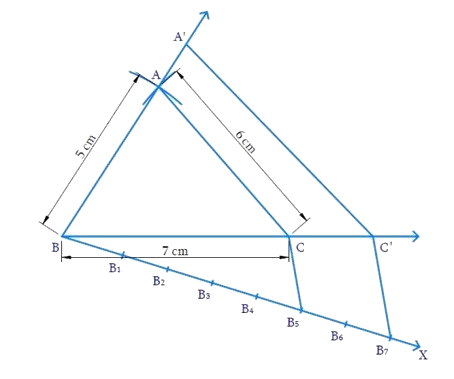Steps of construction:

(i) Draw $${{BC = 7}}\,{\rm{cm}}$$ with $$B$$ and $$C$$ as centres and radii $$5\, \rm{cm}$$ and $$6 \,\rm{cm}$$ respectively. Draw arcs to intersect at $$A.$$ $$\,\Delta {{ABC}}$$ is obtained.

(ii) Draw ray $$BX$$ making $$\,\angle {{CBX}}$$ acute.

(iii) Mark $$7$$ points (greater of $$7$$ and $$5$$ in \begin{align}\frac{7}{5}\end{align} ) $${{{B}}_{{I}}}{{,}}\,{{{B}}_{{2}}}{{,}}\,{{ \ldots \ldots \ldots }}{{{B}}_{{7}}}$$ on $$BX$$ such that $$\,{{B}}{{{B}}_{{I}}}{{ = }}{{{B}}_{{I}}}{{{B}}_{{2}}}{{ = \ldots \ldots \ldots \ldots = }}{{{B}}_{{6}}}{{{B}}_{{7}}}$$

(iv) Join $${{{B}}_{{5}}}$$ (smaller of $$7$$ and $$5$$ in \begin{align}\frac{7}{5}\end{align} and so the $$5^\rm{th}$$ point) to $$C$$ and draw $${{{B}}_{{7}}}{{C'}}$$ parallel to $${{{B}}_{{5}}}{{C}}$$ intersecting the extension of $$BC$$ at  $$C'$$.

(v) Through $${{C'}}$$ draw $${{C'A'}}$$ parallel to $$CA$$ to meet the extension of $$BA$$ at $$A’.$$

Now, $$\,{{\Delta A'}}\,{{B'}}\,{{C'}}$$ is the required triangle similar to $$\,{{\Delta ABC}}$$ where

\begin{align}\frac{{{{BA'}}}}{{{{BA}}}}{{ = }}\frac{{{{C'A'}}}}{{{{CA}}}}{{ = }}\frac{{{{BC'}}}}{{{{BC}}}}{{ = }}\frac{{{7}}}{{{5}}}\end{align}

Proof:

In $$\Delta {B}{B}_{7}{C',}\;{B}_{3}{C}$$ is parallel to $${B}_{7}{C'}$$

Hence by Basic proportionality theorem,

\begin{align}\frac{{{{{B}}_{{6}}}{{{B}}_{{7}}}}}{{{{B}}{{{B}}_{{5}}}}}&{{ = }}\frac{{{{CC'}}}}{{{{BC}}}}\\&{{ = }}\frac{{{2}}}{{{5}}}\end{align}

Adding $$1$$,

\begin{align}\frac{{{{CC'}}}}{{{{BC}}}}{{ + 1}}& = \frac{{{2}}}{{{5}}}{{ + 1}}\\\frac{{{{BC + CC'}}}}{{{{BC}}}}{{ }}&=\frac{{{7}}}{{{5}}}\\\frac{{{{BC'}}}}{{{{BC}}}}{{ }}&= \frac{{{7}}}{{{5}}}\end{align}

Consider $${{\Delta BAC}}$$ and $${{\Delta BA'C'}}$$

$$\angle {{ABC}} = \angle {{A'BC'}}$$ (Common)

$$\angle \text{BCA}\!\!\text{ }=\angle \text{BC}\!'\text{A}\!\!'\!$$ (Corresponding angles $$∵$$ $$\text{CA}||\,\text{C }\!\!'\!\!\text{ }A'\$$ )

$$\angle {{BAC}} = \angle {{BA'C'}}$$ (Corresponding angles)

By AAA axiom, $$\Delta {{BAC}} \sim \Delta {{BA'C'}}$$

$$∴$$  Corresponding sides are proportional

Hence,

\begin{align}\frac{{{{BA'}}}}{{{{BA}}}}{{ = }}\frac{{{{C'A'}}}}{{{{CA}}}}{{ = }}\frac{{{{BC'}}}}{{{{BC}}}}{{ = }}\frac{{{7}}}{{{5}}}\end{align}

## Chapter 11 Ex.11.1 Question 4

Construct an isosceles triangle whose base is $$8 \;\rm{cm}$$ and altitude $$4 \;\rm{cm}$$ and then another triangle whose sides are \begin{align}1\frac{1}{2} \end{align} times the corresponding sides of the isosceles triangle.

#### What is known?

Base and altitude of an isosceles triangle and the ratio of corresponding sides of $$2$$ triangles.

Construction.

#### Reasoning:

• Draw the line segment of base $$8 \;\rm{cm}$$. Draw perpendicular bisector of the line. Mark a point on the bisector which measures $$4 \;\rm{cm}$$ from the base. Connect this point from both the ends.
• Then draw another line which makes an acute angle with the given line. Divide the line into $$m + n$$ parts where $$m$$ and $$n$$ are the ratio given.
• Two triangles are said to be similar if their corresponding angles are equal. They are said to satisfy Angle-Angle-Angle (AAA) Axiom.
• Basic proportionality theorem states that, “If a straight line is drawn parallel to a side of a triangle, then it divides the other two sides proportionally".

#### Steps: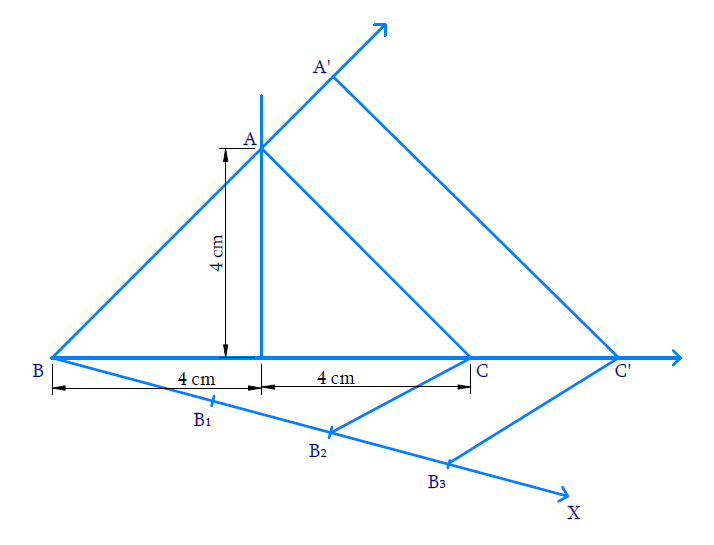Steps of construction:

(i) Draw $$\,{{BC = 8}}\,{\rm{cm}}{{.}}$$ Through $$D,$$ the mid-point of $$BC,$$ draw the perpendicular to $$BC$$ and cut an arc from $$D$$ on it such that $${{DA = 4}}\,{{cm}}{{.}}$$ Join $$BA$$ and $$CA.$$ $${{\Delta ABC}}$$ is obtained.

(ii) Draw the ray $$BX$$ so that $$\angle {{CBX}}$$ is acute.

(iii) Mark $$3$$ \begin{align}\left( {3{{ }} > {{ }}\;2\text{ in}\,\,1\frac{1}{2} = \,\frac{3}{2}} \right)\end{align}  points $${{{B}}_{{I}}}{{,}}\,{{{B}}_{{2}}}{{,}}\,{{{B}}_{{3}}}$$ on BX such that $${{B}}{{{B}}_{{1}}}{{ = }}{{{B}}_{{1}}}{{{B}}_{{2}}}{{ = }}{{{B}}_{{2}}}{{{B}}_{{3}}}$$

(iv) Join $${B}_{2}$$ ($$\;2^\rm{nd}$$  point $$∵ \;2<3$$) to C and draw $${B}_{3} {C}^{\prime}$$ parallel to $${B}_{2} {C}$$ intersect $$BC$$ extended at $$C’$$.

(v)  Through $$C’$$ draw $${{C'A'}}$$ parallel to $$CA$$ to intersect $$BA$$ extended to $$A’.$$

Now, $${{\Delta A'BC'}}$$ is the required triangle similar to $$\,{{\Delta ABC}}$$ where

\begin{align}\frac{{{{BA'}}}}{{{{BA}}}}{{ = }}\frac{{{{C'A'}}}}{{{{CA}}}}{{ = }}\frac{{{{BC'}}}}{{{{BC}}}}{{ = }}\frac{{{3}}}{{{2}}}\end{align}

Proof:

In $${{\Delta B}}{{{B}}_{{3}}}{{C',}}\,\,{{{B}}_{{2}}}{{C}}\,\,{{||}}\,\,{{{B}}_{{3}}}{{C'}}$$ ,

Hence by Basic proportionality theorem,

\begin{align}\frac{{{{{B}}_{{2}}}{{{B}}_{{3}}}}}{{{{B}}{{{B}}_{{2}}}}}{{ = }}\frac{{{{CC'}}}}{{{{BC}}}}{{ = }}\frac{{{1}}}{{{2}}}\end{align}

Adding $$1$$,

\begin{align}\frac{{{{CC'}}}}{{{{BC}}}}{{ + 1}}&= \frac{{{1}}}{{{2}}}{{ + 1}}\\\frac{{{{BC + CC'}}}}{{{{BC}}}}{{ }}&=\frac{{{3}}}{{{2}}}\\\frac{{{{BC'}}}}{{{{BC}}}}{{}}&= \frac{{{3}}}{{{2}}}\end{align}

Consider $$\,{{\Delta BAC }}\,{{and }}\,{{\Delta BA'C'}}$$

$$\angle {{ABC}}\, = \,\angle {{A'BC'}}$$ (Common)

$$\angle {BCA}=\angle {BC' } { A '}$$ (Corresponding angles $$\therefore \mathrm{CA} \| \mathrm{C}^{\prime} \mathrm{A}^{\prime}$$

$$\angle {{BAC}}\, = \,\angle {{BA'C'}}$$ (Corresponding angles)

By AAA axiom, $${{\Delta BAC \sim\Delta BA'C'}}$$

$$∴$$ Corresponding sides are proportional

Hence,

\begin{align}\frac{{{{BA'}}}}{{{{BA}}}}{{ = }}\frac{{{{BC'}}}}{{{{BC}}}}{{ = }}\frac{{{{CA'}}}}{{{{CA}}}}{{ = }}\frac{{{3}}}{{{2}}}\end{align}

## Chapter 11 Ex.11.1 Question 5

Draw a triangle $$ABC$$ with side $${{BC = 6 \,\rm{cm,}\;AB = 5\,\rm{cm}}}$$ and $$\angle {{ABC = 6}}{{{0}}^0}.$$  Then construct a triangle whose sides are \begin{align}\frac{3}{2}\end{align} of the corresponding sides of the triangle $$ABC.$$

#### What is known?

$$2$$ sides and the angle between them and the ratio of corresponding sides of $$2$$ triangles.

Construction.

#### Reasoning:

• Draw the triangle with the given conditions.
• Then draw another line which makes an acute angle with the base line. Divide the line into $$m + n$$ parts where $$m$$ and $$n$$ are the ratio given.
• Two triangles are said to be similar if their corresponding angles are equal. They are said to satisfy Angle-Angle-Angle (AAA) Axiom.
• Basic proportionality theorem states that, “If a straight line is drawn parallel to a side of a triangle, then it divides the other two sides proportionally".

#### Steps: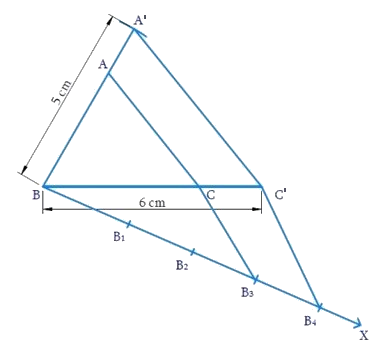Steps of constructions:

(i) Draw $${{BC = 6 \,\rm{cm}}}.$$ At $$B,$$ make $$\angle {{CBY = }}\,{{60^\circ }}$$ and cut an arc at $$A$$ so that $$BA = 5 \,\rm{cm}.$$ Join $$\,{{AC}}\,,\,\,{{\Delta ABC}}$$ is obtained.

(ii) Draw the ray $$BX$$ such that $$\angle {{CBX}}$$ is acute.

(iii) Mark $$4$$ ($${4} > 3$$ in $$\frac{3}{4}$$) points $${{{B}}_1},{{{B}}_{{2}}},\,{{{B}}_{{3}}},\,{{{B}}_{{4}}}$$ on $$BX$$ such that $${{B}}{{{B}}_{{1}}}{{ = }}{{{B}}_{{1}}}{{{B}}_{{2}}}{{ = }}{{{B}}_{{2}}}{{{B}}_{{3}}}{{ = }}{{{B}}_{{3}}}{{{B}}_{{4}}}$$

(iv) Join $${{{B}}_{{4}}}$$ to $$C$$ and draw $${{{B}}_{{3}}}{{C'}}$$ parallel to $${{{B}}_{{4}}}{{C}}$$ to intersect $$BC$$ at $${{C'}}{{.}}$$

(v) Draw $${{C'A'}}$$ parallel to $$CA$$ to intersect $$BA$$ at $$A’.$$

Now, $$\,{{\Delta A'BC'}}$$ is the required triangle similar to $${{\Delta ABC}}$$ where

\begin{align}\frac{{{{BA'}}}}{{{{BA}}}}{{ = }}\frac{{{{BC'}}}}{{{{BC}}}}{{ = }}\frac{{{{C'A'}}}}{{{{CA}}}}{{ = }}\frac{{{3}}}{{{4}}}\end{align}

Proof:

In $${{\Delta }}\,{{B}}{{{B}}_4}{{C'}}\,\,,\,\,{{{B}}_3}{{C' || }}{{{B}}_4}{{C}}$$

Hence by Basic proportionality theorem,

\begin{align}\frac{{{{{B}}_{{3}}}{{{B}}_{{4}}}}}{{{{B}}{{{B}}_{{3}}}}}&= \frac{{{{C'C}}}}{{{{BC'}}}}= \frac{{{1}}}{{{3}}}\\\frac{{{{C'C}}}}{{{{BC'}}}}{{ + 1}}& = \frac{{{1}}}{{{3}}}{{ + 1 \quad \text{(Adding 1)}}}\\\frac{{{{C'C + BC'}}}}{{{{BC'}}}}& = \frac{{{4}}}{{{3}}}\\\frac{{{{BC}}}}{{{{BC'}}}}& =\frac{{{4}}}{{{3}}}\,\, {\rm{(or)}} \,\frac{{{{BC'}}}}{{{{BC}}}} =\frac{{{3}}}{{{4}}}\end{align}

Consider $${{\Delta BA'C'}}\,\,{{and}}\,\,{{\Delta BAC}}$$

\begin{align} & \angle \text{A }\!\!'\!\!\text{ BC }\!\!'\!\!\text{ }=\angle \text{ABC}={{60}^{\circ }} \\ & \angle \text{BCA }\!\!'\!\!\text{ }=\angle \text{BCA}\,\,\\&\left( \text{Corresponding}\,\text{angles}\because \,\text{CA}||\text{CA} \right) \\ & \angle \text{BA }\!\!'\!\!\text{ C }\!\!'\!\!\text{ }=\angle \text{BAC}\\&\left( \text{Corresponding}\,\text{angles} \right) \\ \end{align}

By AAA axiom, $${{\Delta BA'C' \sim \Delta BAC}}$$

Therefore corresponding sides are proportional,

\begin{align}\frac{{{{BC'}}}}{{{{BC}}}}{{ = }}\frac{{{{BA'}}}}{{{{BA}}}}{{ = }}\frac{{{{C'A'}}}}{{{{CA}}}}{{ = }}\frac{{{3}}}{{{4}}}\end{align}

## Chapter 11 Ex.11.1 Question 6

Draw a triangle ABC with side \begin{align}{{BC }} = {{ }}7\,{\rm{ cm,}}\,\,\angle {{B }} = {{ }}45^\circ ,\,\,\angle {{A }} = {{ }}105^\circ .\end{align} Then, construct a triangle whose sides are \begin{align}\frac{4}{3}\end{align} times the corresponding sides of \begin{align}\Delta{ ABC}\end{align}.

#### What is known?

One side and $$2$$ angles of a triangle and the ratio of corresponding sides of $$2$$ triangles.

Construction.

#### Reasoning:

• Draw the triangle with the given conditions.
• Then draw another line which makes an acute angle with the base line. Divide the line into $$m + n$$ parts where $$m$$ and $$n$$ are the ratio given.
• Two triangles are said to be similar if their corresponding angles are equal. They are said to satisfy Angle-Angle-Angle (AAA) Axiom.
• Basic proportionality theorem states that, “If a straight line is drawn parallel to a side of a triangle, then it divides the other two sides proportionally".

#### Steps: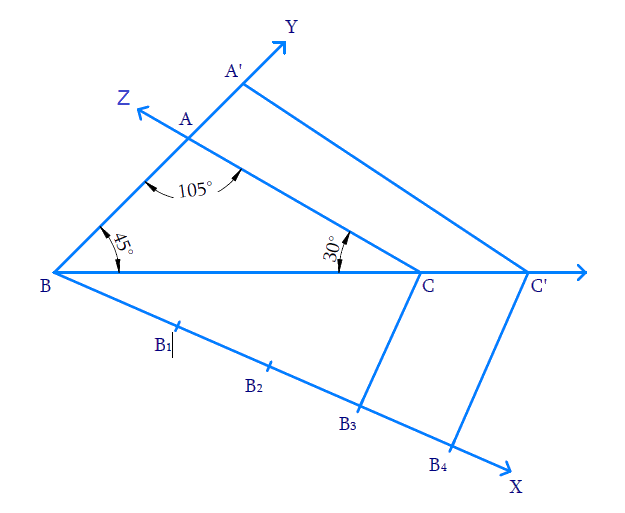Steps of construction:

(i) Draw $${{BC = 7}}\,{\rm{cm}}{{.}}$$ At $$B,$$ make an angle $$\angle {{CBY}} = {45^ \circ }$$ and at $$C,$$ make $$\angle {{BCZ}} = 30^\circ \,\,\left[ {{{180}^ \circ } - \left( {{{45}^ \circ } + {{105}^ \circ }} \right)} \right]$$ . Both $$BY$$ and $$CZ$$ intersect at $$A$$ and thus $${{\Delta ABC}}$$ is constructed.

(ii) Draw the ray $$BX$$ so that $$\angle {{CBX}}$$ is acute.

(iii) Mark $$4$$ \begin{align}\left( {4{{ }} > {{ }}3\,{\rm{ in}}\,\,\frac{4}{3}} \right)\end{align} points $${{{B}}_{{1}}}{{,}}{{{B}}_{{2}}}{{,}}{{{B}}_{{3}}}{{,}}{{{B}}_{{4}}}$$ on BX such that $${{B}}{{{B}}_{{1}}}{{ = }}{{{B}}_{{1}}}{{{B}}_{{2}}}{{ = }}{{{B}}_{{2}}}{{{B}}_{{3}}}{{ = }}{{{B}}_{{3}}}{{{B}}_{{4}}}$$

(iv) Join $$B_3$$ (third point on $$BX,\;3\, < 4$$ in \begin{align}\frac{4}{3}\end{align}) to $$C$$ and draw $${{{B}}_{{4}}}{{C'}}$$ parallel to $$BC$$ such that $$C’$$ lies on the extension of $$BC.$$

(v) Draw $$C’A’$$ parallel to $$CA$$ to intersect the extension of $$BA$$ at $$A’.$$

Now, $$\Delta {{A'BC'}}$$ is the required triangle similar to $${{\Delta ABC}}$$ where,

\begin{align}\frac{{{{BA'}}}}{{{{BA}}}}{{ = }}\frac{{{{BC'}}}}{{{{BC}}}}{{ = }}\frac{{{{C'A'}}}}{{{{CA}}}}{{ = }}\frac{{{4}}}{{{3}}}\end{align}

Proof:

In $${{\Delta B}}{{{B}}_{{4}}}{{C',}}\,\,{{{B}}_{{3}}}{{C}}\,\,{{||}}\,\,{{{B}}_{{4}}}{{C'}}$$

Hence by Basic proportionality theorem,

\begin{align}\frac{{{{{B}}_{{3}}}{{{B}}_{{4}}}}}{{{{B}}{{{B}}_{{3}}}}}&= \frac{{{{CC'}}}}{{{{BC}}}}{{ = }}\frac{{{1}}}{{{3}}}\\\frac{{{{CC'}}}}{{{{BC}}}}{{ + 1 }}&=\frac{{{1}}}{{{3}}}{{ + 1}}\,\quad{\rm{(Adding}}\,{{1)}}\\\frac{{{{BC + CC'}}}}{{{{BC}}}}& = \frac{{{4}}}{{{3}}}\\\frac{{{{BC'}}}}{{{{BC}}}}& = \frac{{{4}}}{{{3}}}\end{align}

Consider $$\Delta BA'C'$$ and  $$\Delta BAC$$

$$\angle {{A'BC'}}\, = \angle {{ABC}} = 45^\circ$$

$$\angle {{BC'A'}} = \angle {{BCA}} = 30^\circ$$ (Correspomding angles as $$\,\,{{CA}}\,{{||}}\,\,{{C'A'}}$$ )

$$\angle {{BA'C'}} = \angle {{BAC}} = 105^\circ$$ (Corresponding angles )

By AAA axiom, $${{\Delta BA'C' \sim \Delta BAC}}$$

Hence corresponding sides are proportional

\begin{align}\frac{{{{BA'}}}}{{{{BA}}}}{{ = }}\frac{{{{BC'}}}}{{{{BC}}}}{{ = }}\frac{{{{C'A'}}}}{{{{CA}}}}{{ = }}\frac{{{4}}}{{{3}}}\end{align}

## Chapter 11 Ex.11.1 Question 7

Draw a right triangle in which the sides (other than hypotenuse) are of lengths $$4 \,\rm{cm}$$ and $$3 \,\rm{cm}$$. Then construct another triangle whose sides are \begin{align}\frac{5}{3}\end{align} times the corresponding sides of the given triangle.

### Solution

What is known?

$$2$$ sides and the angle between them and the ratio of corresponding sides of $$2$$ triangles.

What is unknown?

Construction.

Reasoning:

• Draw the triangle with the given conditions.
• Then draw another line which makes an acute angle with the base line. Divide the line into $$m + n$$ parts where $$m$$ and $$n$$ are the ratio given.
• Two triangles are said to be similar if their corresponding angles are equal. They are said to satisfy Angle-Angle-Angle (AAA) Axiom.
• Basic proportionality theorem states that, “If a straight line is drawn parallel to a side of a triangle, then it divides the other two sides proportionally".

Steps: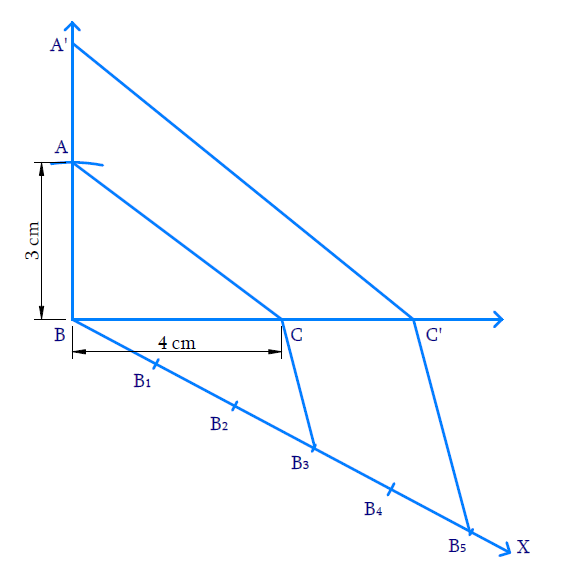Steps of constructions:

(i) Draw $${{BC = 4\, \rm{cm}}}{{.}}$$ At  $$B,$$ make an angle $$\angle {{CBY}} = {90^ \circ }$$ and mark $$A$$ on $$BY$$ such that $${{BA = 3 }}\,{\rm{cm}}{{.}}$$ Join $$A$$ to $$C.$$ Thus $${{\Delta ABC}}$$ is constructed.

(ii) Draw the ray $$BX$$ so that $$\angle {{CBX}}$$ is acute.

(iii) Mark $$5$$ ($$5 > 3$$ in $$\frac{5}{3}$$ ) points $${{{B}}_{{I}}}{{,}}\,{{{B}}_{{2}}}{{,}}\,{{{B}}_{{3}}}{{,}}\,{{{B}}_{{4}}}{{,}}{{{B}}_{{5}}}$$ on $$BX$$ so that

\begin{align}&{{B}}{{{B}}_{{1}}}\\=&{{{B}}_{{1}}}{{{B}}_{{2}}}\\=&{{{B}}_{{2}}}{{{B}}_{{3}}}\\=&{{{B}}_{{3}}}{{{B}}_{{4}}}\\=&{{{B}}_{{4}}}{{{B}}_{{5}}}\end{align}

(iv) Join$${B}_{3}$$ ($$3^\rm{rd}$$ point on $$BX$$ as $$3<5$$) to $$C$$ and draw $${{{B}}_{{5}}}{{C'}}$$ parallel to $${{{B}}_{{3}}}{{C}}$$ so that $${{C'}}$$ lies on the extension of $$BC.$$

(v) Draw $${{C'A'}}$$ parallel to $$CA$$ to intersect of the extension of $$BA$$ at $$A’.$$

Now $${{\Delta BA'C'}}$$ is the required triangle similar to $${{\Delta BAC}}$$ where

\begin{align}\frac{{{{BA'}}}}{{{{BA}}}}{{ = }}\frac{{{{BC'}}}}{{{{BC}}}}{{ = }}\frac{{{{C'A'}}}}{{{{CA}}}}{{ = }}\frac{{{5}}}{{{3}}}\end{align}

Proof:

In $${{\Delta B}}{{{B}}_{{3}}}{{C',}}\,\,{{{B}}_{{3}}}{{C}}\,\,{{||}}\,\,{{{B}}_{{3}}}{{{C}}^{{1}}}$$

Hence by Basic proportionality theorem,

\begin{align} \frac{{{{B}}_{{3}}}{{{B}}_{{5}}}}{{B}{{{B}}_{{3}}}}&{=}\frac{{CC }\!\!'\!\!{ }}{{BC}}{=}\frac{{2}}{{3}} \\ \frac{{CC }\!\!'\!\!{ }}{{BC}} {+1}&=\frac{{2}}{{3}}{+1}\qquad \text{(Adding}\,\,{1)} \\ \frac{{CC }\!\!'\!\!{ +BC}}{{BC}} &{=}\frac{{5}}{{3}} \\ \frac{{BC }\!\!'\!\!{ }}{{BC}}&{=}\frac{{5}}{{3}} \end{align}

Consider $${{\Delta BAC}}$$ and $${{\Delta BA'C'}}$$

$$\angle {{ABC}} = \angle {{A'BC'}} = 90^\circ$$

$$\angle {{BCA}} = \angle {{BC'A'}}$$ (Corresponding angles as $${{CA}}\,\,\,{{||}}\,\,{{C'A'}}$$ )

$$\angle {{BAC}} = \angle {{BA'C'}}$$

By AAA axiom, $${{\Delta BAC}}\,\sim\,{{\Delta BA'C'}}$$

Therefore corresponding sides are proportional,

Hence,

\begin{align}\frac{{{{BA'}}}}{{{{BA}}}}{{ = }}\frac{{{{BC'}}}}{{{{BC}}}}&{{ = }}\frac{{{{C'A'}}}}{{{{CA}}}}\\&{{ = }}\frac{{{5}}}{{{3}}}\end{align}

Ncert Class 10 Exercise 11.1
Ncert Solutions For Class 10 Maths Chapter 11 Exercise 11.1

Related Sections
Related Sections